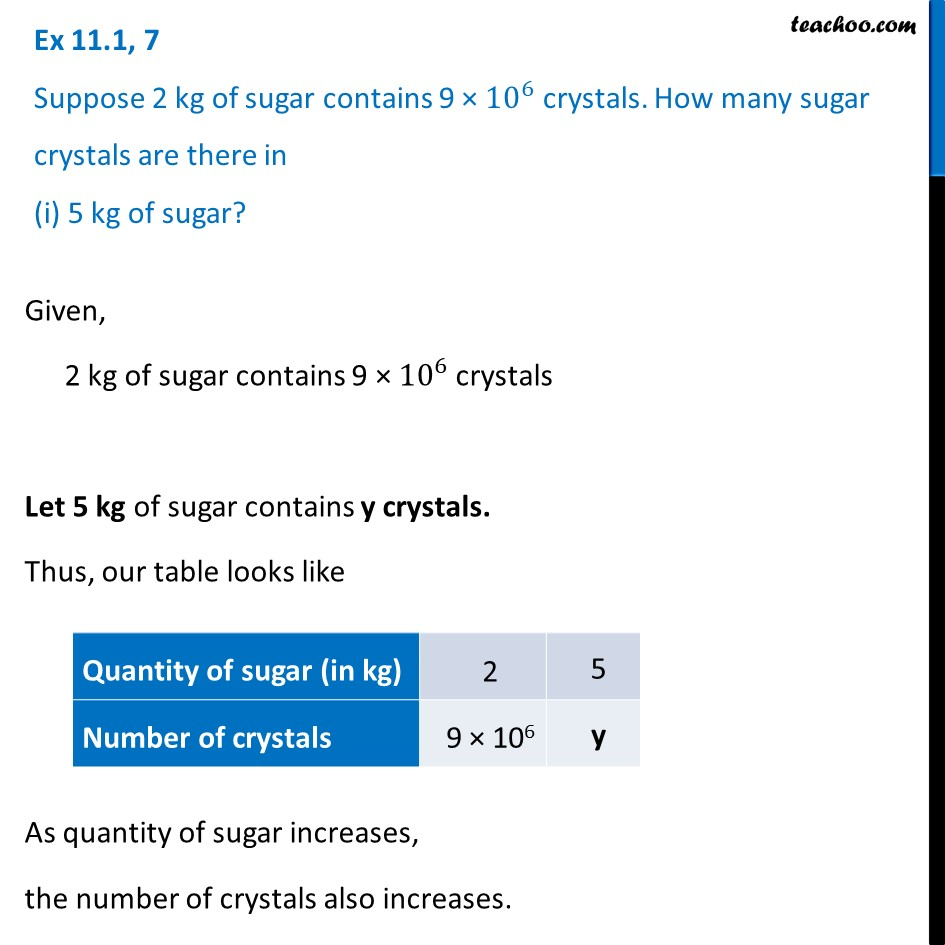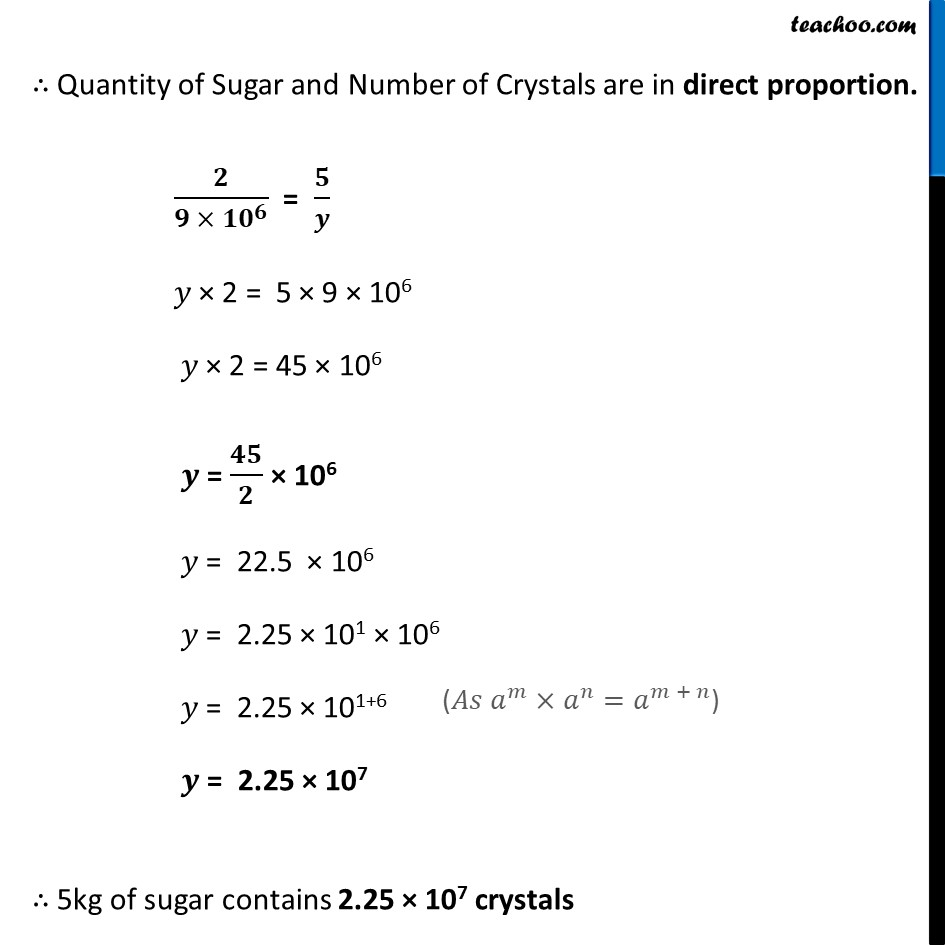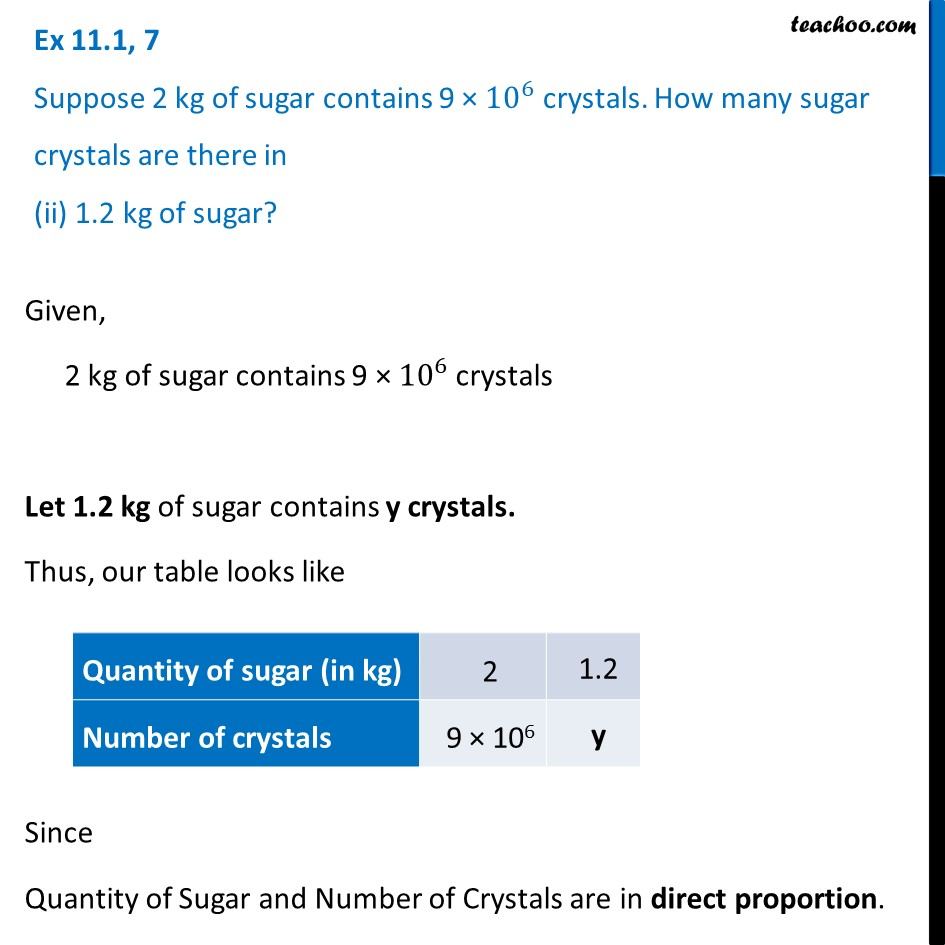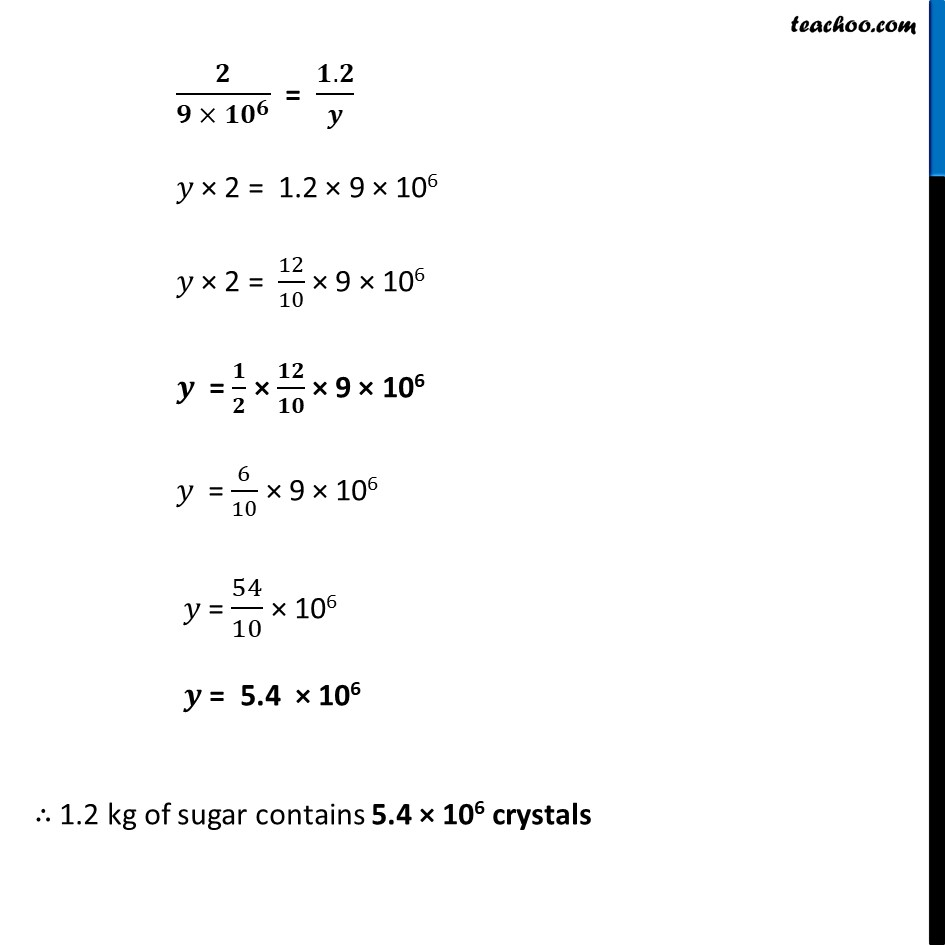Ex 11.1

Chapter 11 Class 8 Direct and Inverse Proportions
Serial order wiseLearn in your speed, with individual attention - Teachoo Maths 1-on-1 Class

### Transcript

Ex 11.1, 7 Suppose 2 kg of sugar contains 9 × 10^6 crystals. How many sugar crystals are there in (i) 5 kg of sugar?Given, 2 kg of sugar contains 9 × 10^6 crystals Let 5 kg of sugar contains y crystals. Thus, our table looks like As quantity of sugar increases, the number of crystals also increases. ∴ Quantity of Sugar and Number of Crystals are in direct proportion. 𝟐/(𝟗 × 〖𝟏𝟎〗^𝟔 ) = 𝟓/𝒚 𝑦 × 2 = 5 × 9 × 106 𝑦 × 2 = 45 × 106 𝒚 = 𝟒𝟓/𝟐 × 106 𝑦 = 22.5 × 106 𝑦 = 2.25 × 101 × 106 𝑦 = 2.25 × 101+6 𝒚 = 2.25 × 107 ∴ 5kg of sugar contains 2.25 × 107 crystals Ex 11.1, 7 Suppose 2 kg of sugar contains 9 × 10^6 crystals. How many sugar crystals are there in (ii) 1.2 kg of sugar?Given, 2 kg of sugar contains 9 × 10^6 crystals Let 1.2 kg of sugar contains y crystals. Thus, our table looks like Since Quantity of Sugar and Number of Crystals are in direct proportion. 𝟐/(𝟗 × 〖𝟏𝟎〗^𝟔 ) = (𝟏.𝟐)/𝒚 𝑦 × 2 = 1.2 × 9 × 106 𝑦 × 2 = 12/10 × 9 × 106 𝒚 = 𝟏/𝟐 × 𝟏𝟐/𝟏𝟎 × 9 × 106 𝑦 = 6/10 × 9 × 106 𝑦 = 54/10 × 106 𝒚 = 5.4 × 106 ∴ 1.2 kg of sugar contains 5.4 × 106 crystals